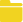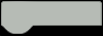# How to Make a Fruit Classification Project with UNIHIKER

UNHIKER supports running machine learning, neural networks, and other AI-related Python libraries. With its ability to process image information, it is perfect for computer vision applications. In this tutorial, we will implement a fruit classification project using UNIHIKER and a USB camera.

Steps:

1. Collect a dataset of images featuring three types of fruits and background for recognition.

2. Train a new model by combining an existing object classification model with the collected image dataset.

3. Employ the generated model to classify the type of fruit.

HARDWARE LIST
1 UNIHIKER
1 USB Camera
1 Gravity: Digital Piranha LED Module - Red
1 Gravity: Digital piranha LED module - Yellow
1 Gravity: Digital Piranha LED Module - Green
1 WS2812-16 RGB LED Ring Lamp

###USB Camera - USB Port

LED Red - P21

LED Yellow - P22

LED Green - P24

*RGB LED Ring Lamp (Optional) - P23

STEP 1
Image Capture with the Camera

In this step, we will capture images of apples, bananas, watermelons, and a white background. These images will be stored in four subdirectories named "01Apple," "02Banana," "03Watermelon," and "04Others" under the "dataset_object_classification" directory.

CODE
``````import cv2
import os
from pinpong.board import *

os.system('mkdir -p dataset_object_classification/01Apple')  # Create a folder to save captured images
os.system('mkdir -p dataset_object_classification/02Banana')
os.system('mkdir -p dataset_object_classification/03Watermelon')
os.system('mkdir -p dataset_object_classification/04Others')

number = input("Please enter the dataset folder number:")
if number == "1":
location = 'dataset_object_classification/01Apple/'
elif number == "2":
location = 'dataset_object_classification/02Banana/'
elif number == "3":
location = 'dataset_object_classification/03Watermelon/'
elif number == "4":
location = 'dataset_object_classification/04Others/'
print(location)

Board().begin()  # Initialize the hardware board

np_16 = NeoPixel(Pin(Pin.P23), 16)  # Create a NeoPixel instance
np_16.clear()  # Clear the display

'''Define functions'''

def lamp_bright():
# Display bright light
lamp_number = 0
for index in range(16):
np_16[lamp_number] = (255, 255, 255)
lamp_number += 1

def lamp_close():
# Turn off the lights
lamp_number = 0
for index in range(16):
np_16[lamp_number] = (0, 0, 0)
lamp_number += 1

lamp_bright()

def get_photo():
# Define the function to take photos (input: image saving location)
cap = cv2.VideoCapture(0)  # Create a VideoCapture object to start the camera (0 represents the default camera)
cap.set(cv2.CAP_PROP_BUFFERSIZE, 1)  # Set the camera buffer to 1 to decrease latency

cv2.namedWindow('window', cv2.WND_PROP_FULLSCREEN)  # Create a window named 'window' and set it to fullscreen
cv2.setWindowProperty('window', cv2.WND_PROP_FULLSCREEN, cv2.WINDOW_FULLSCREEN)  # Set the window to fullscreen

count = 0

while cap.isOpened():
# Loop to read each frame

h, w, c = frame.shape  # Get the shape of the image (height, width, channels)
w1 = h * 240 // 320
x1 = (w - w1) // 2
frame = frame[:, x1:x1 + w1]  # Crop the image
frame = cv2.resize(frame, (240, 320))  # Resize the image to match the board

cv2.imshow("window", frame)  # Display the image in the window

key = cv2.waitKey(1)  # Delay 1ms between frames

if key & 0xFF == ord('b'):  # Press 'b' to exit
lamp_close()
break
elif key & 0xFF == ord('a'):  # Press 'a' to save the image and exit
if count < 100:
cv2.imwrite(location + str(count) + ".jpg", frame)  # Save the image as a file
count += 1
else:
print("image saved")

cap.release()  # Release the camera
cv2.destroyAllWindows()  # Close all windows

get_photo()  # Call the get_photo function to capture images
``````

To collect images using the program, follow these steps:

1. Run the program and enter 1 in the terminal to specify that the captured images will be stored in the "01Apple" directory.2. Aim the camera at an apple or a picture of an apple. Press the 'A' button on UNIHIKER to capture photos.Make sure to capture the apple from different angles. You can hold the 'A' button on to take continuous photos.3. When the terminal displays "image saved," it indicates that 100 images have been successfully collected. You can adjust the number of images according to your preference. More images will result in higher accuracy, while fewer images will make the program run faster.4. To exit the camera view, press the 'B' button of UNIHIKER. At this point, you have collected a dataset of apple images.5. Run the program again, repeat the same process by entering 2, 3, or 4 in the terminal to collect images of bananas, watermelons, and the background, respectively.STEP 2
Train a Classification Model using TensorFlow

Run the following program and wait for the UNIHIKER to train automatically. The training time may vary from 1 to 5 minutes. Once completed, the terminal will display "Model saved!" and an "object_classification_model.h5" model file will be generated in the current directory.

CODE
``````# Import the TensorFlow deep learning framework
import tensorflow as tf
# Import the Keras deep learning library
from tensorflow import keras
# Import the data processing library
import numpy as np

# Model Training: Train the collected image dataset using a neural network
# Run the program and wait for the computer to train automatically. The training time may vary from 1 to 5 minutes. Once completed, the terminal will display "Model saved!" and the "object_classification_model.h5" model file will be generated in the current directory.

# Data Preprocessing
# Prepare the images in a format that can be input to the pre-trained model
# Define the dataset path
train_dir = 'dataset_object_classification'

# ImageDataGenerator is a Keras image generator used to preprocess image data
# mobilenet_v2.preprocess_input is the image preprocessing method for mobilenet_v2, used to prepare the image in a format suitable for the mobilenet_v2 model
datagen = keras.preprocessing.image.ImageDataGenerator(preprocessing_function=keras.applications.mobilenet_v2.preprocess_input)

# The flow_from_directory method of the image generator generates batches of data for model training
train_batches = datagen.flow_from_directory(
directory=train_dir,      # directory: Path to the target folder. The target folder contains multiple subfolders, each representing a class and containing images of that class.
shuffle=True,             # shuffle: Whether to shuffle the data. Set to True to shuffle the order of data processing within each subfolder, otherwise, the data will be processed in alphabetical order based on the image names in each subfolder.
target_size=(96,96),      # target_size: The target size to resize all images.
batch_size=10             # batch_size: The batch size, which represents the number of data in each batch. During preprocessing, the data will be processed in batches.
)

# train_batches returns the input data and label data

# Freeze the Pretrained Model

# The base model used here is mobilenet_v2 with an input image size of 224x224
base_model.trainable = False                                 # Freeze the pre-trained model

# The model here does not include the output layer, only the input layer and hidden layers
# After freezing, the weights and other parameters will not be changed
# The frozen model acts as a feature extractor with only the input layer

# Create the Neural Network

# Instantiate a neural network model
model = keras.Sequential(name="object_classification_model")

# Create the input layer
model.add(base_model)                                   # Set the input layer as the pre-trained model with an input size of 224x224
# Create the hidden layer
model.add(keras.layers.Dense(100, activation='relu'))   # Create a hidden layer with 100 neurons
# Create the output layer
model.add(keras.layers.Dense(4, activation='softmax'))  # Set the output layer for 4-class classification

# # View the model structure
# print("Model structure:")
# model.summary()

# Train the Model

# Set the parameters
model.compile(
loss='categorical_crossentropy',
metrics=['accuracy']
)

# Train the model
print("Start training:")
model.fit(train_batches, epochs=5)  # Train for 5 epochs
print("Training completed!")

# Save the model
model.save("object_classification_model.h5")
print("Model saved!")
``````
STEP 3
Fruit Classification

To view the live video streaming on the UNIHIKER screen and display the classification results across four categories, while lighting up the corresponding LEDs, please execute the following program:

CODE
``````'''Display real-time video streaming on the Pyboard screen and perform live predictions'''

# Import TensorFlow deep learning framework
import tensorflow as tf
# Import Keras deep learning library
from tensorflow import keras
# Import OpenCV computer vision library
import cv2
# Import data processing library
import numpy as np
# Import hardware control library
from pinpong.board import *

Board().begin()               # Initialize
led21 = Pin(Pin.P21, Pin.OUT) # Initialize pin as digital output
led22 = Pin(Pin.P22, Pin.OUT) # Initialize pin as digital output
led24 = Pin(Pin.P24, Pin.OUT) # Initialize pin as digital output
# Create an instance of NeoPixel
np_16 = NeoPixel(Pin(Pin.P23), 16) # Pin 23, 16 LEDs
np_16.clear() # Clear the display
'''Define utility functions'''
# Display different colored lights
def lamp_bright(): # Bright light - white
lamp_number = 0
for index in range(16):
np_16[lamp_number] = (255,255,255)
lamp_number = (lamp_number + 1)
def lamp_close(): # Turn off lights
lamp_number = 0
for index in range(16):
np_16[lamp_number] = (0,0,0)
lamp_number = (lamp_number + 1)
lamp_bright()

# Import the object classification model
print("Importing the model...")
model_name = "object_classification_model.h5"
print("{} model imported successfully".format(model_name))

# Image preprocessing
def preprocess_img(frame):
img = tf.image.resize(frame, (96, 96))                 # Resize image
img_array = keras.preprocessing.image.img_to_array(img)  # Convert image to array
img_array = tf.expand_dims(img_array, 0)                 # Add an additional dimension to match the model input
img_array = img_array/255.                               # Normalize
return img_array                                         # Return preprocessed image data

# Draw classification result
res = frame                                        # Get a frame of the image
box_startpoint = (30,10)                           # Define coordinates
class_names = ["Apple","Banana","Watermelon",""]    # Define classification results

# Draw a histogram
res = cv2.rectangle(res,
(0,0),
(240,35),
(255,255,255),
-1)
# Draw the predicted result below the screen as "predicted result :"+
res = cv2.putText(res,str(class_names[predictions.numpy().argmax()]),
(0,25), # coor
cv2.FONT_HERSHEY_SIMPLEX, 1,
(0, 255, 12),
2,
cv2.LINE_4)
# ZhiXinDu = model.predict(img_array)[model.predict(img_array).argmax()]
res = str(class_names[predictions.numpy().argmax()])
return res

# Initialize the screen
window_name = 'frame'
cap = cv2.VideoCapture(0)            # Get camera image
cap.set(cv2.CAP_PROP_FRAME_WIDTH, 240)
cap.set(cv2.CAP_PROP_FRAME_HEIGHT, 320)
cap.set(cv2.CAP_PROP_BUFFERSIZE, 1)  # Set the number of frames in the internal buffer
cv2.namedWindow(window_name, cv2.WND_PROP_FULLSCREEN)
cv2.setWindowProperty(window_name, cv2.WND_PROP_FULLSCREEN, cv2.WINDOW_FULLSCREEN)

while True:
# Capture frames from the camera
ret, frame = cap.read() # ret is True or False, indicating whether the image was captured, frame represents the current captured frame
h, w, c = frame.shape # Record the shape of the image, representing height, width, and channels
w1 = h*240//320
x1 = (w-w1)//2
frame = frame[:, x1:x1+w1] # Crop the image
frame = cv2.resize(frame, (240, 320)) # Adjust the image size to match the Pyboard screen
img_array = preprocess_img(frame)         # Preprocess the frame
predictions = model.predict(img_array)    # Model prediction (overall probabilities)
predictions = tf.nn.softmax(predictions)  # Output prediction results
new_frame = add_data(frame,predictions)   # Draw classification result for each frame
# frame=cv2ImgAddText(frame,new_frame, (10, 30),(0, 255, 0), 30)
print(new_frame)

# Display the image
cv2.imshow('frame',frame)

# Press the 'A' key on the keyboard to exit the program
if cv2.waitKey(1) & 0xFF == ord('b'):
lamp_close()
break

if new_frame == "Apple": #  ["Apple","Banana","Watermelon",""]
led21.write_digital(1)
else:
led21.write_digital(0)
if new_frame == "Banana":
led22.write_digital(1)
else:
led22.write_digital(0)
if new_frame == "Watermelon":
led24.write_digital(1)
else:
led24.write_digital(0)
if new_frame == "":
led21.write_digital(0)
led22.write_digital(0)
led24.write_digital(0)

# Clear the display
cap.release()
cv2.destroyAllWindows()
``````
STEP 4
Structure Design

To ensure a stable light environment for improved recognition results, we have designed a 3D-printed structure that incorporates a LED ring around the camera and securely positions the camera at a specific height. You can find the 3D print module provided below.Cam Stand.zip 723KB Download(11)